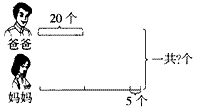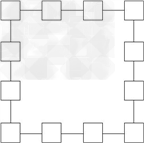• 试题检索 :
• 教材版本 :
• 课本 :
• 题型 :
• 难易度 :

[ ]
A、40只
B、50只
C、80只

[ ]
A、63
B、51
C、61

32+28+72○131                      87-63○125÷5
621÷9○69                            4×12○46-2

8△2=8+9=17
5△3=5+6+7=18
4△6=4+5+6+7+8+9=39
11△4=（    ）
1△x=5050，x=（    ）。21可以写成几个连续的数相加的形式，如21=10+11；33也可以写成几个连续数相加的形式，如33=10+11+12。用这种方法完成下列各式：125=（    ）+（    ），69=（    ）+（    ）+（    ）。